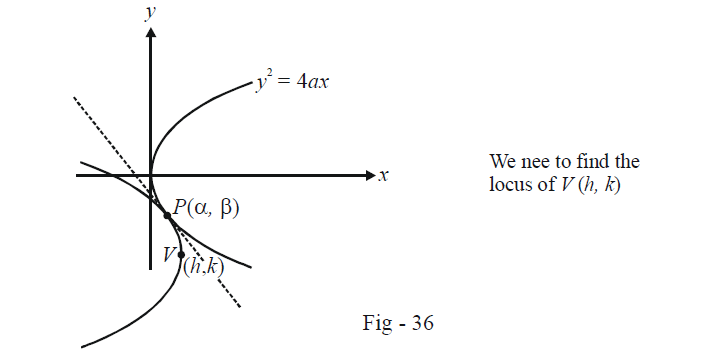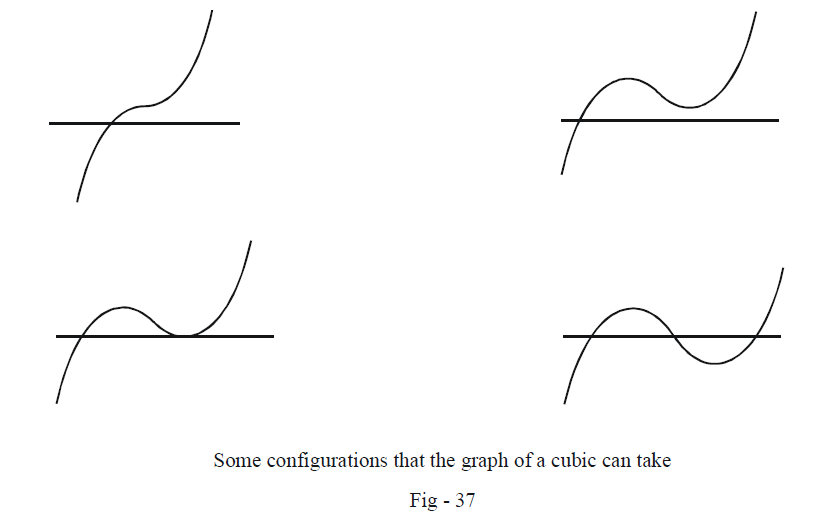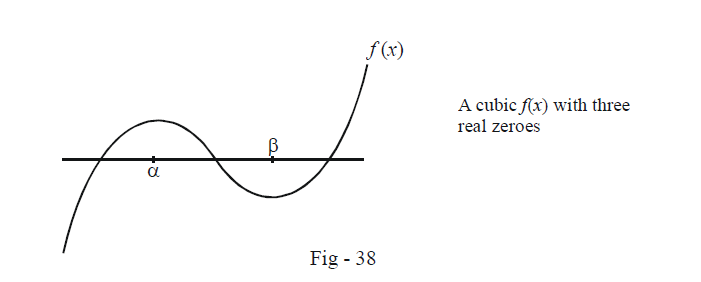# Parabolas Set 2

Go back to  'SOLVED EXAMPLES'

Example – 3

Considera fixed parabola $${y^2} = 4ax.$$ A variable butequal parabola having its axis parallel to the axis of the fixed parabola,moves so that it always touches the fixed parabola. What is the locus of thevertex of the moving parabola ?

Solution: By saying that two parabolas are equal, we can infer that the length of their latus-rectums will the same. It should be obvious that for an equal parabola to touch $${y^2} = 4ax,$$ it must open towards the left (the axisof the variable parabola is parallel to the x-axis.):Let the point of contact of the two parabolas be $$P(\alpha ,\,\beta ).$$ Note that at P the two parabolas will have a common tangent.

We have assumed the vertex of the variable parabola to be V (h, k). Thus the equation of this variable parabola is

${(y - k)^2} = - 4a(x - h) \qquad \dots \left( 1 \right)$

Now, the tangent at $$P(\alpha ,\,\beta){\rm{ }}\;to\;{\rm{ }}{y^2} = 4ax$$ will be

$\beta y = 2a(x + \alpha ) \qquad \dots \left( 2 \right)$

The tangent at $$P(\alpha ,\,\beta )$$ to the variableparabola will be (using (1)),

$(y - k)(\beta - k) = - 2a(x - h + \alpha -h) \qquad \dots \left( 3 \right)$

In writing (3), we have implicitly used a translation of the axis to (h, k) ;verify that (3) is indeed correct. (3) can be written in standard form as

$2ax + (\beta - k)y + 2a(\alpha - 2h) - k(\beta - k) = 0 \qquad \dots (4)$

Now note that (2) and (4) are in fact the same line, so that

$\frac{{2a}}{{2a}}= \frac{{ - \beta }}{{\beta - k}} = \frac{{2a\alpha }}{{2a(\alpha - 2h) -k(\beta - k)}}$

$$\alpha \;and\;\beta$$ can easily be eliminated from these relations to obtain a relation between h and k as

${k^2} = 8ah$

Thus,the required locus of V is

${y^2} = 8ax$

which is a parabola with the same vertex as the original parabola, and the same orientation, as one might have intuitively expected.

Example – 4

Find the condition for all the three normals drawn from a given point P(h, k) to the parabola $${y^2} = 4ax$$ to be real and distinct. Assume a > 0.

Solution: As discussed a lot of times earlier, the given requirement is equivalent to the equation

$k = mh - 2am - a{m^3}$

having three real and distinct roots

A cubic will always have at least one real root. Here are some configurations that the graph of a cubic can take (assuming the coefficient of $${m^3}$$ is positive):Since we want three real roots, we focus on the last graph above. Observe that it has two extremas, say at $$\alpha{\rm{ }}\;{\rm{and}}\;{\rm{ }}\beta$$.Thus, $$f'(x) = 0$$ must have two distinct real roots (since $$f'(\alpha )= f'(\beta ) = 0).$$ Also, we must have $$f(\alpha )f(\beta) < 0.$$ Convince yourself that these two constraints will ensure that the cubic f(x) will have three real and distinct zeroes.

We use this for the current cubic $$f(m)= a{m^3} + (2a - h)m + k$$

• $$f'(m) = 0$$ has two real and distinct roots

\begin{align}&\Rightarrow \quad f'(m) = 3a{m^2} + (2a - h)\\\\&\Rightarrow \quad f'(m) = 0{\rm{ }}\;for\;{\rm{ }}m = \pm \sqrt {\frac{{h - 2a}}{{3a}}} \\\\&\Rightarrow \quad h - 2a > 0{\rm{ }}\;or\;{\rm{ }}h > 2a\end{align}

The two real and distinct roots are

$\alpha = \sqrt {\frac{{h - 2a}}{{3a}}} {\rm{}}\;and\;{\rm{ }}\beta = - \sqrt {\frac{{h - 2a}}{{3a}}} \qquad \dots \left( 1 \right)$

• $$f(\alpha )f(\beta ) < 0$$

\begin{align}&\Rightarrow \qquad (a{\alpha ^3} + (2a - h)\alpha + k)(\alpha {\beta ^3} + (2a - h)\beta + k)< 0\\\\&\Rightarrow \qquad {a^2}{(\alpha \beta )^3} + a(2a - h)\alpha \beta ({\alpha ^2} + {\beta ^2}) +ak({\alpha ^3} + {\beta ^3}) + {k^2} < 0\end{align}

Simplifying this using (1), we obtain

$27a{k^2} < 4{(h - 2a)^3}$

Thus,if we are to be able to draw three real and distinct normals form P(h, k) to $${y^2} = 4ax,$$ h and k must satisfy

$h > 2a{\rm{ }}\;\text{and}\;{\rm{ }}27a{k^2} < 4{(h -2a)^3}$

Learn from the best math teachers and top your exams

• Live one on one classroom and doubt clearing
• Practice worksheets in and after class for conceptual clarity
• Personalized curriculum to keep up with school